PhD Student in the OXCSML and Torr-Vision groups

### An Introduction to PySPPL

pysppl_walkthrough_1

# PySPPL: Introduction¶

Python SPPL is a probabilistic programming language that is built for enabling one to perform inference in probabilistic programs that require gradient based inference algorithms. As if-else statements within probabilistic programs that use gradient based inference, take on two different forms. On one hand hand if-else have the usual programmer interpretation, but on the other, when random variables are placed inside of the predicate, or the body of an if-else expression, then we must treat those variables with care as discontinuities arise at point of indecision. We must ensure that at this indecision boundary the discontinuity, with respect to space it is in and the measure imposed on it, has a measure of zero.

Our framework was built to ensure that certain criteria in the compiled output of a directed acyclic graphical model are met. The language is extensible enough for one to add their own custom built potentials; which could be either log joint densities or loss functions, within the framework. There is a higher layer inference framework that takes advantage of PySPPL called pyfo, we are actively developing this. The corresponding paper is called Discontinuous Hamiltonian Monte Carlo for Probabilistic Programs.

In this walk-through we shall go through the basics of how to write a model and how to compile the model. In the following walk-through we shall show how to use custom density functions, how add other distributions within the framework and how to take advantage of the translation rules that are embedded within the language.

## PySPPL imports¶

In :
%matplotlib inline
from pyppl import compile_model
from pyppl.utils.core import create_network_graph, display_graph


## A statistical model with basic control-flow¶

For fun, let us add some contextual data: Whether or not Alice decides to go to space is highly dependent upon the number of points Alice collects, which is dependent upon a draw from a centered normal distribution, $x_1$. If she draws a number greater than 0, then we observe that Alice gets 1.5 points and the likelihood she goes to space, given the 1.5 points, is distributed by $\mathcal{N}(1.5~|~x_1,1)$. However, if she draws a number less than or equal to 0, then her likelihood is quite different. She gains 1 point, but the likelihood that the distribution is centered around 1, is dependent upon a random draw from a categorical distribution that returns a 0 with probability 0.1, 1 with probability 0.2, and 2 with probability 0.7. If we find that the value of the $x_1$ after the inference is greater than zero, then Alice goes to space, else she stays on Earth :-(

$$x_1 \sim \mathcal{N}(0,1)$$ $$x_2 \sim \mathcal{Cat}(0.1, 0.2, 0.7)$$ we observe our data ("points") $$y_1 = 1.5$$ $$y_2 = 1$$

likelihood terms $$y_1 = 1.5~|~x_1 = \mathcal{N}(y_1~|~x_1,~1)$$ $$y_2 = 1~|~x_2 = \mathcal{N}(y_2~|~x_2,~1)$$

In :
model_if_clojure="""
(let [x1 (sample (normal 0 1))
x2 (sample (categorical [0.1 0.2 0.7]))
y1 1.5
y2 1]
(if (> x1 0)
(observe (normal x1 1) y1)
(observe (normal x2 1) y2))
[x1 x2])
"""

compiled_clojure = compile_model(model_if_clojure, language='clojure')


The compiler takes the above code and transforms it into a model class, from which the user can manipulate the program and interface with an inference engine.

As can be seen above, the language consists of some special syntax, one being sample(<distribution_name>(param1, param2m, ...))

which generates the priors for the variables of interest, and the second being observe(<distribution name>(param1, param2, ...), <observation/data>)

which generates the likelihood given the observed data. The definitions given here are for when writing models in python, but examples of what the statements look like in clojure is given in the example above.

Finally, if-expressions, whilst on the surface look like usual if-statements, are interpreted in a special way in PySPPL. If the predicate, in the example above this is (> x1 0), contains any sampled variables, then within PySPPL a specific key-value pair is generated for that if-statement, so that those variables can be controlled in a special way.

This is especially important when using gradient based inference algorithms, as the conditions within the predicates can create measure zero discontinuities. When using algorithms such as Hamiltonian Monte Carlo (HMC) for performing inference in models that are of a low dimensionality with a suitably low number of discontinuities, one may not notice a difference in the outcome of the inference result. If they have the ground truth available. However, using HMC on such models is completely and utterly statistically wrong. But the HMC algorithm for such a model would run without hindrance.

This is dangerous for two reasons. One, If probabilistic programming languages and systems are supposed to deal with these subtleties whilst letting the modeller focus on modelling, then we are letting down a large group of the target users, who may not truly understand these subtleties. Two, the use of gradient based inference with control flow is poorly understood. Whilst point two, is re-iterating point one to some extent, it is important to understand, that a lack of understanding about a particular inference technique is detrimental to the setup of the probabilistic programming language and system. This is one of the reasons behind PySPPL, a simple language with inbuilt constraints to avoid these issue, yet is flexible enough to be integrated into other probabilistic programming systems.

Here is a print out of the model class for the above model:

In :
print(compiled_clojure.code)

# 2018-06-18 09:42:24.644173
import torch
import torch.distributions as dist

class Model():

def __init__(self, vertices: set, arcs: set, data: set, conditionals: set):
super().__init__()
self.vertices = vertices
self.arcs = arcs
self.data = data
self.conditionals = conditionals

def __repr__(self):
V = '\n'.join(sorted([repr(v) for v in self.vertices]))
A = ', '.join(['({}, {})'.format(u.name, v.name) for (u, v) in self.arcs]) if len(self.arcs) > 0 else '  -'
C = '\n'.join(sorted([repr(v) for v in self.conditionals])) if len(self.conditionals) > 0 else '  -'
D = '\n'.join([repr(u) for u in self.data]) if len(self.data) > 0 else '  -'
graph = 'Vertices V:\n{V}\nArcs A:\n  {A}\n\nConditions C:\n{C}\n\nData D:\n{D}\n'.format(V=V, A=A, C=C, D=D)
graph = '#Vertices: {}, #Arcs: {}\n'.format(len(self.vertices), len(self.arcs)) + graph
return graph

def gen_cond_bit_vector(self, state):
result = 0
for cond in self.conditionals:
result = cond.update_bit_vector(state, result)
return result

def gen_cond_vars(self):
return [c.name for c in self.conditionals]

def gen_cont_vars(self):
return [v.name for v in self.vertices if v.is_continuous and not v.is_conditional and v.is_sampled]

def gen_disc_vars(self):
return [v.name for v in self.vertices if v.is_discrete and v.is_sampled]

def gen_if_vars(self):
return [v.name for v in self.vertices if v.is_conditional and v.is_sampled and v.is_continuous]

def gen_log_pdf(self, state):
log_pdf = 0
dst_ = dist.Normal(loc=0, scale=1)
log_pdf = log_pdf + dst_.log_pdf(state['x30001'])
dst_ = dist.Categorical(probs=[0.1, 0.2, 0.7])
log_pdf = log_pdf + dst_.log_pdf(state['x30002'])
state['cond_30003'] = (state['x30001'] > 0)
dst_ = dist.Normal(loc=state['x30001'], scale=1)
if state['cond_30003']:
log_pdf = log_pdf + dst_.log_pdf(state['y30004'])
dst_ = dist.Normal(loc=state['x30002'], scale=1)
if not state['cond_30003']:
log_pdf = log_pdf + dst_.log_pdf(state['y30005'])
return log_pdf

def gen_prior_samples(self):
state = {}
dst_ = dist.Normal(loc=0, scale=1)
state['x30001'] = dst_.sample()
dst_ = dist.Categorical(probs=[0.1, 0.2, 0.7])
state['x30002'] = dst_.sample()
state['cond_30003'] = (state['x30001'] > 0)
dst_ = dist.Normal(loc=state['x30001'], scale=1)
state['y30004'] = 1.5
dst_ = dist.Normal(loc=state['x30002'], scale=1)
state['y30005'] = 1
return state

def get_arcs(self):
return self.arcs

def get_arcs_names(self):
return [(u.name, v.name) for (u, v) in self.arcs]

def get_conditions(self):
return self.conditionals

def get_vars(self):
return [v.name for v in self.vertices if v.is_sampled]

def get_vertices(self):
return self.vertices

def get_vertices_names(self):
return [v.name for v in self.vertices]

def is_torch_imported(self):
import sys
print('torch' in sys.modules)
print(torch.__version__)
print(type(torch.tensor))
import inspect
print(inspect.getfile(torch))



We can change the models imported by changing the arguments in the compile_model function. By specification to our current application the compiler automatically imports pytorch. This can be easily removed by going into ppl_graph_codegen.py file and then removing the if not has_dist block.

In :
compile_clojure = compile_model(model_if_clojure, language='clojure',imports='import matplotlib as mpl \nimport numpy as np ')
print(compile_clojure.code[:121])

# 2018-06-18 09:55:22.261447
import torch
import torch.distributions as dist
import matplotlib as mpl
import numpy as np


## Printing the Graph G(V,E) of the statistical model.¶

Each vertex of the graph is an object, with a set of attributes. These attributes describe the relationships between the vertex and other vertices. In addition to this, the attributes contain information about the observables and latent variables at each vertex.

In :
print(compile_clojure)

#Vertices: 4, #Arcs: 2
Vertices V:
Vertex x30001 [Sample]
Name:           x30001
Ancestors:
Cond-Ancs.:
Dist-Args:      {'loc': '0', 'scale': '1'}
Dist-Code:      dist.Normal(0, 1)
Dist-Name:      Normal
Dist-Type:      DistributionType.CONTINUOUS
Sample-Size:    1
Vertex x30002 [Sample]
Name:           x30002
Ancestors:
Cond-Ancs.:
Dist-Args:      {'probs': '[0.1, 0.2, 0.7]'}
Dist-Code:      dist.Categorical([0.1, 0.2, 0.7])
Dist-Name:      Categorical
Dist-Type:      DistributionType.DISCRETE
Sample-Size:    1
Vertex y30004 [Observe]
Name:           y30004
Ancestors:      x30001
Conditions:     cond_30003=True
Cond-Ancs.:     x30001
Cond-Nodes:     cond_30003
Dist-Args:      {'loc': "state['x30001']", 'scale': '1'}
Dist-Code:      dist.Normal(state['x30001'], 1)
Dist-Name:      Normal
Dist-Type:      DistributionType.CONTINUOUS
Sample-Size:    1
Observation:    1.5
Vertex y30005 [Observe]
Name:           y30005
Ancestors:      x30002
Conditions:     cond_30003=False
Cond-Ancs.:     x30001
Cond-Nodes:     cond_30003
Dist-Args:      {'loc': "state['x30002']", 'scale': '1'}
Dist-Code:      dist.Normal(state['x30002'], 1)
Dist-Name:      Normal
Dist-Type:      DistributionType.CONTINUOUS
Sample-Size:    1
Observation:    1
Arcs A:
(x30001, y30004), (x30002, y30005)

Conditions C:
Condition
Name:         cond_30003
Ancestors:    x30001
Condition:    (state['x30001'] > 0)
Function:     state['x30001']
Op:           >

Data D:
-



We can see from the graph that $x_2$ is only dependent on the observation $y_2$, whereas $x_1$ can be affected by both observations.

## An example of an independent Categorical model¶

In :
model_categorical = """
(let[z (sample (categorical [0.7 0.15 0.15]))
z1 (sample (categorical [0.1 0.5 0.4]))
z2 (sample (categorical [0.2 0.2 0.6]))]
z z1 z2)"""

In :
compiled_clojure = compile_model(model_categorical, language='clojure')
# print(compiled_clojure.code)
vertices = compiled_clojure.vertices


### Plotting the depndence graph¶

In :
create_network_graph(vertices=vertices)
display_graph(vertices=vertices);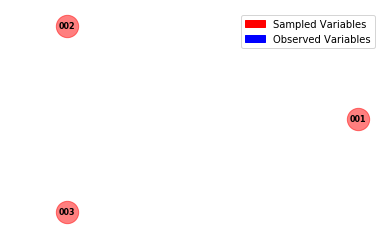As you can see, in this model there are no dependencies between the different latent variables.

## A Hidden Markov Model¶

In a HMM the goal is to infer the hidden states. In doing so we aim to explain how our observations are produced, as the underlying assumption is that the hidden state directly affects the observation produced. This is a very short description, of a very powerful tool. See here for more details on HMMs.

In :
model_hmm_clojure="""
(defn data [n]
(let [points (vector 0.9 0.8 0.7 0.0 -0.025
5.0 2.0 0.1 0.0 0.13
0.45 6.0 0.2 0.3 -1.0 -1.0)]
(get points n)))

;; Define the init, transition, and observation distributions
(defn get-init-params []
(vector (/ 1. 3.) (/ 1. 3.) (/ 1. 3.)))

(defn get-trans-params [k]
(nth (vector (vector 0.1  0.5  0.4 )
(vector 0.2  0.2  0.6 )
(vector 0.7 0.15 0.15 )) k))

(defn get-obs-dist [k]
(nth (vector (normal -1. 1.)
(normal  1. 1.)
(normal  0. 1.)) k))

;; Function to step through HMM and sample latent state
(defn hmm-step [n states]
(let [next-state (sample (categorical (get-trans-params (last states))))]
(observe (get-obs-dist next-state) (data n))
(conj states next-state)))

;; Loop through the data
(let [init-state (sample (categorical (get-init-params)))]
(loop 16 (vector init-state) hmm-step))

"""


### Plotting the graphical model¶

In :
compiled_clojure = compile_model(model_hmm_clojure, language='clojure')
vertices = compiled_clojure.vertices
create_network_graph(vertices=vertices)
display_graph(vertices=vertices);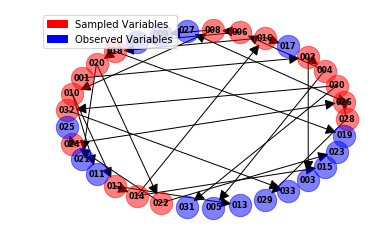# PySPPL for python based models¶

PySPPLs design allows one to use it with many different languages, we so far have built an interface for both python and clojure code. It is also important to note that the number of distributions one can add to PySPPL is boundless, as we will show in future walk-throughs.

## Bayesian linear regression model¶

The aim of inference in this model is to infer the equation of the line $x = y*s + b$ (this is written to match the notation below. In general one would see his written as $y = mx +c$).

$$slope \sim \mathcal{N}(0, 10)$$ $$bias \sim \mathcal{N}(0, 10)$$

Observations $$y = [(1.,2.), (2.1,3.9), (3.,5.3)]$$ Constructing the equation of the predicted curve $$x_n = slope \times y[0,:] + bias$$ The likelihood $$y = y[1,:]~|~x_n = \mathcal{N}(y[1,:]~|~x_n,~\mathbf{I})$$

## The Python SPPL code¶

In :
model_lr_python = """import torch
slope = sample(normal(torch.tensor(0.0), torch.tensor(10.0)))
bias  = sample(normal(torch.tensor(0.0), torch.tensor(10.0)))
y  = torch.tensor([[1.0, 2.1], [2.0, 3.9], [3.0, 5.3]])
xn = slope*data[:,0] + bias # y  = mx + c
observe(normal(xn, torch.ones(len(xn))),data[:,1])

[slope, bias]
"""

compiled_python = compile_model(model_lr_python, language='python')


## The Graph¶

In :
print(compiled_python)

#Vertices: 3, #Arcs: 2
Vertices V:
Vertex x30001 [Sample]
Name:           x30001
Ancestors:
Cond-Ancs.:
Dist-Args:      {'loc': 'torch.tensor(0.0)', 'scale': 'torch.tensor(10.0)'}
Dist-Code:      dist.Normal(torch.tensor(0.0), torch.tensor(10.0))
Dist-Name:      Normal
Dist-Type:      DistributionType.CONTINUOUS
Sample-Size:    1
Orig. Name:     slope
Vertex x30002 [Sample]
Name:           x30002
Ancestors:
Cond-Ancs.:
Dist-Args:      {'loc': 'torch.tensor(0.0)', 'scale': 'torch.tensor(10.0)'}
Dist-Code:      dist.Normal(torch.tensor(0.0), torch.tensor(10.0))
Dist-Name:      Normal
Dist-Type:      DistributionType.CONTINUOUS
Sample-Size:    1
Orig. Name:     bias
Vertex y30003 [Observe]
Name:           y30003
Ancestors:      x30002, x30001
Conditions:
Cond-Ancs.:
Cond-Nodes:
Dist-Args:      {'loc': "((state['x30001'] * state['data'][:,0]) + state['x30002'])", 'scale': "torch.ones(len(((state['x30001'] * state['data'][:,0]) + state['x30002'])))"}
Dist-Code:      dist.Normal(((state['x30001'] * state['data'][:,0]) + state['x30002']), torch.ones(len(((state['x30001'] * state['data'][:,0]) + state['x30002']))))
Dist-Name:      Normal
Dist-Type:      DistributionType.CONTINUOUS
Sample-Size:    1
Observation:    state['data'][:,1]
Arcs A:
(x30002, y30003), (x30001, y30003)

Conditions C:
-

Data D:
-



## The dependency graph¶

With python models we can plot the user defined label names.

In :
vertices = compiled_python.vertices
create_network_graph(vertices=vertices)
display_graph(vertices=vertices);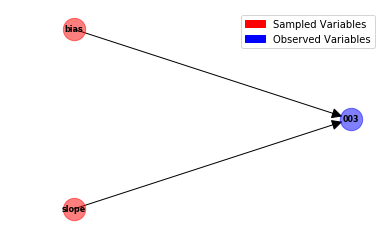# Models in pure python¶

We don't need to import torch, we can actually write models in pure python, for example:

In :
model_purepython="""mean = sample(poisson(3))
x= sample(gamma(mean,1))
y= 10
observe(normal(x,5), y)
"""
compiled_python = compile_model(model_purepython, language='python')


## An Example of the Graph G(V,E)¶

In :
print(compiled_python)

#Vertices: 3, #Arcs: 2
Vertices V:
Vertex x30001 [Sample]
Name:           x30001
Ancestors:
Cond-Ancs.:
Dist-Args:      {'lam': '3'}
Dist-Code:      dist.Poisson(3)
Dist-Name:      Poisson
Dist-Type:      DistributionType.DISCRETE
Sample-Size:    1
Orig. Name:     mean
Vertex x30002 [Sample]
Name:           x30002
Ancestors:      x30001
Cond-Ancs.:
Dist-Args:      {'alpha': "state['x30001']", 'beta': '1'}
Dist-Code:      dist.Gamma(state['x30001'], 1)
Dist-Name:      Gamma
Dist-Type:      DistributionType.CONTINUOUS
Sample-Size:    1
Orig. Name:     x
Vertex y30003 [Observe]
Name:           y30003
Ancestors:      x30002
Conditions:
Cond-Ancs.:
Cond-Nodes:
Dist-Args:      {'loc': "state['x30002']", 'scale': '5'}
Dist-Code:      dist.Normal(state['x30002'], 5)
Dist-Name:      Normal
Dist-Type:      DistributionType.CONTINUOUS
Sample-Size:    1
Observation:    10
Arcs A:
(x30001, x30002), (x30002, y30003)

Conditions C:
-

Data D:
-


In :
vertices = compiled_python.vertices
create_network_graph(vertices=vertices)
display_graph(vertices=vertices);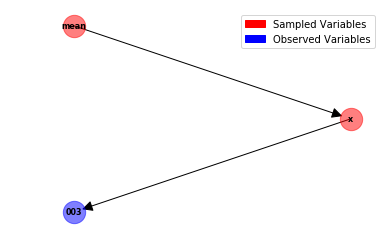## Gaussian Mixture model¶

In this model the goal of the inference procedure is to infer the unknown means that describe the observations. Gaussian mixtures assume that the data can be modelled by a collection of Gaussian distributions (of course this is by no means always true).

In :
model_gmm2 = """
import torch

means  = 2
samples  = 10
y = [-2.0, -2.5, -1.7, -1.9, -2.2, 1.5, 2.2, 3.0, 1.2, 2.8,
-1.7, -1.3,  3.2,  0.8, -0.9, 0.3, 1.4, 2.1, 0.8, 1.9]
ys = torch.tensor([y])
pi = torch.tensor(0.5*torch.ones(samples,means))
mus = sample(normal(torch.zeros(means,1), 2*torch.ones(means,1)))

zn = sample(categorical(pi), sample_size=2)

for i in range(samples):
index = (zn == i).nonzero()
observe(normal(mus[i]*torch.ones(len(index),1), 2*torch.ones(len(index),1)), ys[index])
"""

compiled_python = compile_model(model_gmm2, language='python')


## The code output¶

In :
print(compiled_python.code)

# 2018-06-15 08:40:37.239078
import torch
import torch.distributions as dist
import torch

class Model():

def __init__(self, vertices: set, arcs: set, data: set, conditionals: set):
super().__init__()
self.vertices = vertices
self.arcs = arcs
self.data = data
self.conditionals = conditionals

def __repr__(self):
V = '\n'.join(sorted([repr(v) for v in self.vertices]))
A = ', '.join(['({}, {})'.format(u.name, v.name) for (u, v) in self.arcs]) if len(self.arcs) > 0 else '  -'
C = '\n'.join(sorted([repr(v) for v in self.conditionals])) if len(self.conditionals) > 0 else '  -'
D = '\n'.join([repr(u) for u in self.data]) if len(self.data) > 0 else '  -'
graph = 'Vertices V:\n{V}\nArcs A:\n  {A}\n\nConditions C:\n{C}\n\nData D:\n{D}\n'.format(V=V, A=A, C=C, D=D)
graph = '#Vertices: {}, #Arcs: {}\n'.format(len(self.vertices), len(self.arcs)) + graph
return graph

def gen_cond_bit_vector(self, state):
result = 0
for cond in self.conditionals:
result = cond.update_bit_vector(state, result)
return result

def gen_cond_vars(self):
return [c.name for c in self.conditionals]

def gen_cont_vars(self):
return [v.name for v in self.vertices if v.is_continuous and not v.is_conditional and v.is_sampled]

def gen_disc_vars(self):
return [v.name for v in self.vertices if v.is_discrete and v.is_sampled]

def gen_if_vars(self):
return [v.name for v in self.vertices if v.is_conditional and v.is_sampled and v.is_continuous]

def gen_log_pdf(self, state):
log_pdf = 0
dst_ = dist.Normal(loc=torch.zeros(2, 1), scale=(2 * torch.ones(2, 1)))
log_pdf = log_pdf + dst_.log_pdf(state['x30001'])
dst_ = dist.Categorical(probs=torch.tensor((0.5 * torch.ones(10, 2))))
log_pdf = log_pdf + dst_.log_pdf(state['x30002'])
dst_ = dist.Normal(loc=(state['x30001'] * torch.ones(len((state['x30002'] == 0).nonzero()), 1)), scale=(2 * torch.ones(len((state['x30002'] == 0).nonzero()), 1)))
log_pdf = log_pdf + dst_.log_pdf(state['y30004'])
dst_ = dist.Normal(loc=(state['x30001'] * torch.ones(len((state['x30002'] == 1).nonzero()), 1)), scale=(2 * torch.ones(len((state['x30002'] == 1).nonzero()), 1)))
log_pdf = log_pdf + dst_.log_pdf(state['y30005'])
dst_ = dist.Normal(loc=(state['x30001'] * torch.ones(len((state['x30002'] == 2).nonzero()), 1)), scale=(2 * torch.ones(len((state['x30002'] == 2).nonzero()), 1)))
log_pdf = log_pdf + dst_.log_pdf(state['y30006'])
dst_ = dist.Normal(loc=(state['x30001'] * torch.ones(len((state['x30002'] == 3).nonzero()), 1)), scale=(2 * torch.ones(len((state['x30002'] == 3).nonzero()), 1)))
log_pdf = log_pdf + dst_.log_pdf(state['y30007'])
dst_ = dist.Normal(loc=(state['x30001'] * torch.ones(len((state['x30002'] == 4).nonzero()), 1)), scale=(2 * torch.ones(len((state['x30002'] == 4).nonzero()), 1)))
log_pdf = log_pdf + dst_.log_pdf(state['y30008'])
dst_ = dist.Normal(loc=(state['x30001'] * torch.ones(len((state['x30002'] == 5).nonzero()), 1)), scale=(2 * torch.ones(len((state['x30002'] == 5).nonzero()), 1)))
log_pdf = log_pdf + dst_.log_pdf(state['y30009'])
dst_ = dist.Normal(loc=(state['x30001'] * torch.ones(len((state['x30002'] == 6).nonzero()), 1)), scale=(2 * torch.ones(len((state['x30002'] == 6).nonzero()), 1)))
log_pdf = log_pdf + dst_.log_pdf(state['y30010'])
dst_ = dist.Normal(loc=(state['x30001'] * torch.ones(len((state['x30002'] == 7).nonzero()), 1)), scale=(2 * torch.ones(len((state['x30002'] == 7).nonzero()), 1)))
log_pdf = log_pdf + dst_.log_pdf(state['y30011'])
dst_ = dist.Normal(loc=(state['x30001'] * torch.ones(len((state['x30002'] == 8).nonzero()), 1)), scale=(2 * torch.ones(len((state['x30002'] == 8).nonzero()), 1)))
log_pdf = log_pdf + dst_.log_pdf(state['y30012'])
dst_ = dist.Normal(loc=(state['x30001'] * torch.ones(len((state['x30002'] == 9).nonzero()), 1)), scale=(2 * torch.ones(len((state['x30002'] == 9).nonzero()), 1)))
log_pdf = log_pdf + dst_.log_pdf(state['y30013'])
return log_pdf

def gen_log_pdf_transformed(self, state):
log_pdf = 0
dst_ = dist.Normal(loc=torch.zeros(2, 1), scale=(2 * torch.ones(2, 1)), transformed=True)
log_pdf = log_pdf + dst_.log_pdf(state['x30001'])
dst_ = dist.Categorical(probs=torch.tensor((0.5 * torch.ones(10, 2))), transformed=True)
log_pdf = log_pdf + dst_.log_pdf(state['x30002'])
dst_ = dist.Normal(loc=(state['x30001'] * torch.ones(len((state['x30002'] == 0).nonzero()), 1)), scale=(2 * torch.ones(len((state['x30002'] == 0).nonzero()), 1)), transformed=True)
log_pdf = log_pdf + dst_.log_pdf(state['y30004'])
dst_ = dist.Normal(loc=(state['x30001'] * torch.ones(len((state['x30002'] == 1).nonzero()), 1)), scale=(2 * torch.ones(len((state['x30002'] == 1).nonzero()), 1)), transformed=True)
log_pdf = log_pdf + dst_.log_pdf(state['y30005'])
dst_ = dist.Normal(loc=(state['x30001'] * torch.ones(len((state['x30002'] == 2).nonzero()), 1)), scale=(2 * torch.ones(len((state['x30002'] == 2).nonzero()), 1)), transformed=True)
log_pdf = log_pdf + dst_.log_pdf(state['y30006'])
dst_ = dist.Normal(loc=(state['x30001'] * torch.ones(len((state['x30002'] == 3).nonzero()), 1)), scale=(2 * torch.ones(len((state['x30002'] == 3).nonzero()), 1)), transformed=True)
log_pdf = log_pdf + dst_.log_pdf(state['y30007'])
dst_ = dist.Normal(loc=(state['x30001'] * torch.ones(len((state['x30002'] == 4).nonzero()), 1)), scale=(2 * torch.ones(len((state['x30002'] == 4).nonzero()), 1)), transformed=True)
log_pdf = log_pdf + dst_.log_pdf(state['y30008'])
dst_ = dist.Normal(loc=(state['x30001'] * torch.ones(len((state['x30002'] == 5).nonzero()), 1)), scale=(2 * torch.ones(len((state['x30002'] == 5).nonzero()), 1)), transformed=True)
log_pdf = log_pdf + dst_.log_pdf(state['y30009'])
dst_ = dist.Normal(loc=(state['x30001'] * torch.ones(len((state['x30002'] == 6).nonzero()), 1)), scale=(2 * torch.ones(len((state['x30002'] == 6).nonzero()), 1)), transformed=True)
log_pdf = log_pdf + dst_.log_pdf(state['y30010'])
dst_ = dist.Normal(loc=(state['x30001'] * torch.ones(len((state['x30002'] == 7).nonzero()), 1)), scale=(2 * torch.ones(len((state['x30002'] == 7).nonzero()), 1)), transformed=True)
log_pdf = log_pdf + dst_.log_pdf(state['y30011'])
dst_ = dist.Normal(loc=(state['x30001'] * torch.ones(len((state['x30002'] == 8).nonzero()), 1)), scale=(2 * torch.ones(len((state['x30002'] == 8).nonzero()), 1)), transformed=True)
log_pdf = log_pdf + dst_.log_pdf(state['y30012'])
dst_ = dist.Normal(loc=(state['x30001'] * torch.ones(len((state['x30002'] == 9).nonzero()), 1)), scale=(2 * torch.ones(len((state['x30002'] == 9).nonzero()), 1)), transformed=True)
log_pdf = log_pdf + dst_.log_pdf(state['y30013'])
return log_pdf.sum()

def gen_prior_samples(self):
state = {}
dst_ = dist.Normal(loc=torch.zeros(2, 1), scale=(2 * torch.ones(2, 1)))
state['x30001'] = dst_.sample()
dst_ = dist.Categorical(probs=torch.tensor((0.5 * torch.ones(10, 2))))
state['x30002'] = dst_.sample(sample_size=2)
state['data_30003'] = [-2.0, -2.5, -1.7, -1.9, -2.2, 1.5, 2.2, 3.0, 1.2, 2.8, -1.7, -1.3, 3.2, 0.8, -0.9, 0.3, 1.4, 2.1, 0.8, 1.9]
dst_ = dist.Normal(loc=(state['x30001'] * torch.ones(len((state['x30002'] == 0).nonzero()), 1)), scale=(2 * torch.ones(len((state['x30002'] == 0).nonzero()), 1)))
state['y30004'] = torch.tensor([state['data_30003']])[(state['x30002'] == 0).nonzero()]
dst_ = dist.Normal(loc=(state['x30001'] * torch.ones(len((state['x30002'] == 1).nonzero()), 1)), scale=(2 * torch.ones(len((state['x30002'] == 1).nonzero()), 1)))
state['y30005'] = torch.tensor([state['data_30003']])[(state['x30002'] == 1).nonzero()]
dst_ = dist.Normal(loc=(state['x30001'] * torch.ones(len((state['x30002'] == 2).nonzero()), 1)), scale=(2 * torch.ones(len((state['x30002'] == 2).nonzero()), 1)))
state['y30006'] = torch.tensor([state['data_30003']])[(state['x30002'] == 2).nonzero()]
dst_ = dist.Normal(loc=(state['x30001'] * torch.ones(len((state['x30002'] == 3).nonzero()), 1)), scale=(2 * torch.ones(len((state['x30002'] == 3).nonzero()), 1)))
state['y30007'] = torch.tensor([state['data_30003']])[(state['x30002'] == 3).nonzero()]
dst_ = dist.Normal(loc=(state['x30001'] * torch.ones(len((state['x30002'] == 4).nonzero()), 1)), scale=(2 * torch.ones(len((state['x30002'] == 4).nonzero()), 1)))
state['y30008'] = torch.tensor([state['data_30003']])[(state['x30002'] == 4).nonzero()]
dst_ = dist.Normal(loc=(state['x30001'] * torch.ones(len((state['x30002'] == 5).nonzero()), 1)), scale=(2 * torch.ones(len((state['x30002'] == 5).nonzero()), 1)))
state['y30009'] = torch.tensor([state['data_30003']])[(state['x30002'] == 5).nonzero()]
dst_ = dist.Normal(loc=(state['x30001'] * torch.ones(len((state['x30002'] == 6).nonzero()), 1)), scale=(2 * torch.ones(len((state['x30002'] == 6).nonzero()), 1)))
state['y30010'] = torch.tensor([state['data_30003']])[(state['x30002'] == 6).nonzero()]
dst_ = dist.Normal(loc=(state['x30001'] * torch.ones(len((state['x30002'] == 7).nonzero()), 1)), scale=(2 * torch.ones(len((state['x30002'] == 7).nonzero()), 1)))
state['y30011'] = torch.tensor([state['data_30003']])[(state['x30002'] == 7).nonzero()]
dst_ = dist.Normal(loc=(state['x30001'] * torch.ones(len((state['x30002'] == 8).nonzero()), 1)), scale=(2 * torch.ones(len((state['x30002'] == 8).nonzero()), 1)))
state['y30012'] = torch.tensor([state['data_30003']])[(state['x30002'] == 8).nonzero()]
dst_ = dist.Normal(loc=(state['x30001'] * torch.ones(len((state['x30002'] == 9).nonzero()), 1)), scale=(2 * torch.ones(len((state['x30002'] == 9).nonzero()), 1)))
state['y30013'] = torch.tensor([state['data_30003']])[(state['x30002'] == 9).nonzero()]
return state

def get_arcs(self):
return self.arcs

def get_arcs_names(self):
return [(u.name, v.name) for (u, v) in self.arcs]

def get_conditions(self):
return self.conditionals

def get_vars(self):
return [v.name for v in self.vertices if v.is_sampled]

def get_vertices(self):
return self.vertices

def get_vertices_names(self):
return [v.name for v in self.vertices]

def is_torch_imported(self):
import sys
print('torch' in sys.modules)
print(torch.__version__)
print(type(torch.tensor))
import inspect
print(inspect.getfile(torch))



## The dependency graph¶

In :
vertices = compiled_python.vertices
create_network_graph(vertices=vertices)
display_graph(vertices=vertices);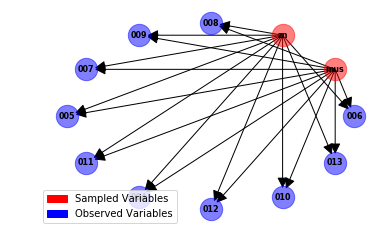## Coal Mining model¶

This model is popular change point detection model in the statistics community. The goal of the model is to infer, whether or not improvements in technology and safety had an impact on reducing the number of coal mining related deaths. See here for more details.

In :
model_coal_mining="""
e = sample(exponential(1))
l = sample(exponential(1))
T = 112
s = sample(uniform(0,10))
y = [4,5,4,1,0,4,3,4,0,6,3,3,4,0,2,6,3,3,5,4,5,3,1,4,4,1,5,5,
3,4,2,5,2,2,3,4,2,1,3,2,1,1,1,1,1,3,0,0,1,0,1,1,0,0,3,1,
0,3,2,2,0,1,1,1,0,1,0,1,0,0,0,2,1,0,0,0,1,1,0,2,2,3,1,1,
2,1,1,1,1,2,4,2,0,0,0,1,4,0,0,0,1,0,0,0,0,0,1,0,0,1,0,0]
for i in range(T-1):
if i+1 < s:
observe(exponential(e), y[i])
else:
observe(exponential(1), y[i])
[e,l,s]
"""
compiled_python = compile_model(model_coal_mining, language='python')


## The dependency graph¶

In :
vertices = compiled_python.vertices
create_network_graph(vertices=vertices)
display_graph(vertices=vertices);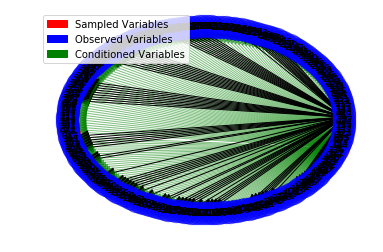I would like to thank Andrea Patanè, Sasha Salter and Saeid Naderiparizi for proof reading the post.